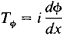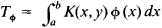[¦self ə¦jȯint ′äp·ə‚rād·ər]
(mathematics)
A linear operator which is identical with its adjoint operator.
McGraw-Hill Dictionary of Scientific & Technical Terms, 6E, Copyright © 2003 by The McGraw-Hill Companies, Inc.
The following article is from The Great Soviet Encyclopedia (1979). It might be outdated or ideologically biased.

(or Hermitian operator), an operator coincident with its adjoint. The theory of self-adjoint operators arose as a generalization of the theory of, for example, integral equations with a symmetric kernel, self-adjoint differential equations, and symmetric matrices. Examples of self-adjoint operators are (1) the operator of multiplication by the independent variable in the space of functions that are defined on the entire number axis and are square integrable and (2) the differentiation operatorin the same space.

If the function K(x,y) is continuous in the square a ≤ x ≤ b,a ≤ y ≤ b and if K(x,y) = K(y, x), then the integral operatoris self-adjoint. The spectrum of a self-adjoint operator lies on the real axis. In quantum mechanics physical quantities have corresponding self-adjoint operators whose spectra give the possible values of these quantities. A self-adjoint operator can be represented as an integral that is the limit of linear combinations of pairwise orthogonal projection operators with real coefficients.